UPSC  >  Introduction: Dice

# Introduction: Dice - CSAT Preparation - UPSC

## What is a Dice?

Dice is a cube. In cube there are 6 faces. Some important points are given below:

• There are 6 faces in the cube - ABCG, GCDE, DEFH, BCDH, AGEF and ABHF.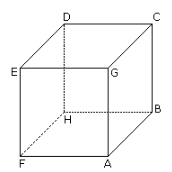• Always four faces are adjacent to one face.
• Opposite of ABCG is DEFH and so on.
• CDEG is the upper face of the cube.
• ABHF is the bottom of the cube.

There are certain rules with the help of these rules question on dice can easily determined.

### Rule No. 1:

Two opposite faces cannot be adjacent to one another.

Example:

Two different positions of a dice are shown below. Which number will appear on the face opposite to the face with number 4?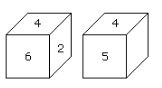Solution:

Faces with four numbers 6, 2, 5 and 3 are adjacent of to the face with No. 4.

Hence the faces with no. 6, 2, 5 and 3 cannot be opposite to the face with no. 4.

Therefore the remaining face with no.1 will be the opposite of the face with no. 4.

### Rule No. 2:

If two different positions of a dice are shown and one of the two common faces is in the same position then of the remaining faces will be opposite to each other.

Example:

Two different positions of a dice are shown below.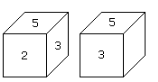Solution:

Here in both shown positions two faces 5 and 3 are common.

The remaining faces are 2 and 4.

Hence the number on the face opposite to the face with number 2 is 4.

### Rule No. 3:

If in two different positions of dice, the position of a common face be the same, then each of the opposite faces of the remaining faces will be in the same position.

Example: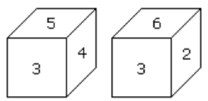Solution:

Here in both positions of common (3) is same.

Therefore, opposite of 5 is 6 and opposite of 4 is 2.

### Rule No. 4:

If in two different positions of a dice, the position of the common face be not the same, then opposite face of the common face will be that which is not shown on any face in these two positions. Besides, the opposite faces of the remaining faces will not be the same.

Example: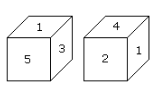Solution:

Here in two positions of a dice the face with number 1 is not in the same position.

The face with number 6 is not shown.

Hence the face opposite to the face with number 1 is 6.

Besides the opposite face of 3 will be the face with number 2 and opposite face to face 5 will be the face with number 1.

The document Introduction: Dice | CSAT Preparation - UPSC is a part of the UPSC Course CSAT Preparation.
All you need of UPSC at this link: UPSC

## FAQs on Introduction: Dice - CSAT Preparation - UPSC

 1. What is a dice?Ans. A dice is a small object, typically cube-shaped, marked with different numbers or symbols on each face. It is commonly used in various games and gambling activities where chance or probability is involved.
 2. How many sides does a traditional dice have?Ans. A traditional dice has six sides, numbered from 1 to 6. Each side represents a different number, and when rolled, the outcome is determined by the number that appears on the top face.
 3. Can a dice have more than six sides?Ans. Yes, dice can have more than six sides. While the traditional dice has six sides, known as a six-sided dice or d6, there are also dice with four sides (d4), eight sides (d8), ten sides (d10), twelve sides (d12), and even twenty sides (d20). These dice are commonly used in various role-playing games.
 4. How do you use a dice in games?Ans. To use a dice in games, you hold it in your hand and give it a good shake. Then, you roll the dice by releasing it from your hand and allowing it to fall onto a flat surface. The outcome is determined by the number or symbol that appears on the top face of the dice after it comes to a stop.
 5. Can dice be used for educational purposes?Ans. Yes, dice can be used for educational purposes. They are often used in classrooms to teach concepts such as probability, mathematical operations, and problem-solving. By incorporating dice into educational activities and games, students can engage in hands-on learning and develop their critical thinking skills.

## CSAT Preparation

197 videos|151 docs|200 tests

## CSAT Preparation

197 videos|151 docs|200 tests

### How to Prepare for UPSC

Read our guide to prepare for UPSC which is created by Toppers & the best Teachers
Signup to see your scores go up within 7 days! Learn & Practice with 1000+ FREE Notes, Videos & Tests.
10M+ students study on EduRev
Track your progress, build streaks, highlight & save important lessons and more!(Scan QR code)
Related Searches

,

,

,

,

,

,

,

,

,

,

,

,

,

,

,

,

,

,

,

,

,

;